Version: v1.1.3

# Sparse Spatial Data Structures

##### note

Prerequisite: please read the Fields, Fields (advanced), and SNodes first.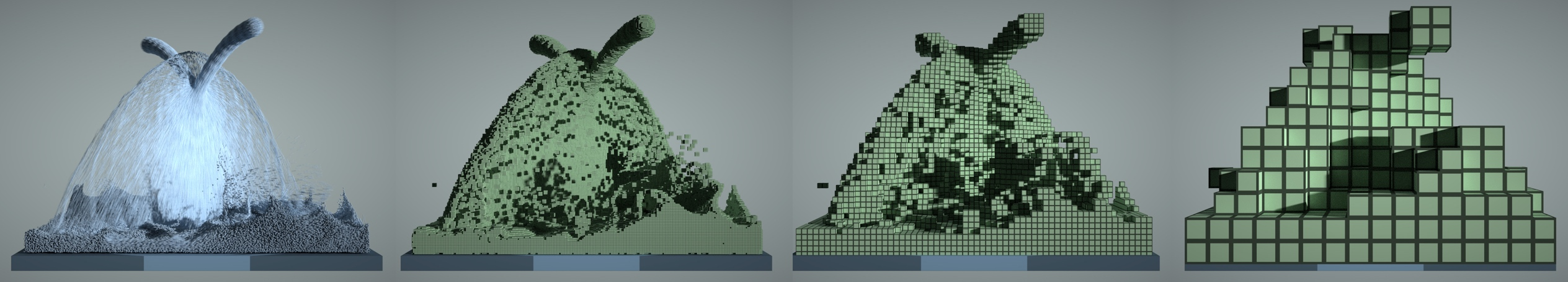Figure: A 3D fluid simulation that uses both particles and grids. Left to right: particles, 1x1x1 voxels, 4x4x4 blocks, 16x16x16 blocks.

## Motivation

High-resolution 2D/3D grids are often needed in large-scale spatial computation, such as physical simulation, rendering, and 3D reconstruction. However, these grids tend to consume a huge amount of memory space and computation if we use dense data structures (see field and field advanced). While a programmer may allocate large dense grids to store spatial data (especially physical quantities such as a density or velocity field), oftentimes, they only care about a small fraction of this dense grid since the rest may be empty space (vacuum or air).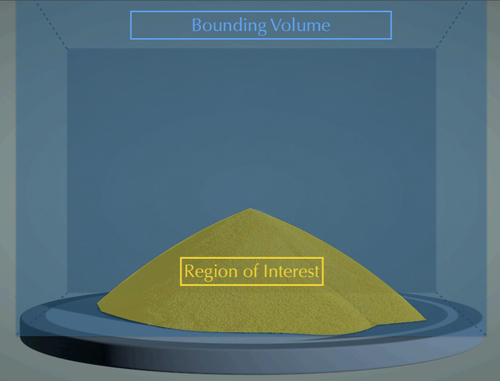For example, the regions of interest in sparse grids shown above may only occupy a small fraction of the whole bounding box. If we can leverage such "spatial sparsity" and focus computation on the regions we care about, we will significantly save storage and computing power.

##### note

The key to leveraging spatial sparsity is replacing dense grids with sparse grids.

The traditional sparse spatial data structures are Quadtrees (2D) and Octrees (3D). Since dereferencing pointers is relatively costly on modern computer architectures, compared to quadtrees and octrees, it is more performance-friendly to use shallower trees with larger branching factors. VDB and SPGrid are such examples. In Taichi, programmers can compose data structures similar to VDB and SPGrid with SNodes. The advantages of Taichi sparse spatial data structures include

1. Access with indices, which just like accessing a dense data structure.
2. Automatic parallelization when iterating.
3. Automatic memory access optimization.
##### note

Backend compatibility: The LLVM backends (CPU/CUDA) and the Metal backend offer the full functionality of computation on sparse spatial data structures.

##### note

Sparse matrices are usually not implemented in Taichi via sparse spatial data structures. See sparse matrix instead.

## Sparse spatial data structures in Taichi

Sparse spatial data structures in Taichi are usually composed of `pointer`, `bitmasked`, `dynamic`, and `dense` SNodes. A SNode tree merely composed of `dense` SNodes is not a sparse spatial data structure.

On a sparse spatial data structure, we consider a pixel, voxel, or a grid node to be active, if it is allocated and involved in the computation. The rest of the grid is simply inactive. In SNode terms, the activity of a leaf or intermediate cell is a boolean value. The activity value of a cell is `True` if and only if the cell is active. When writing to an inactive cell, Taichi automatically activates it. Taichi also provides manual manipulation of the activity of a cell, see Explicitly manipulating and querying sparsity.

##### note

Reading an inactive pixel returns zero.

### Pointer SNode

pointer.py
``x = ti.field(ti.f32)block = ti.root.pointer(ti.ij, (4,4))pixel = block.dense(ti.ij, (2,2))pixel.place(x)@ti.kerneldef activate():    x[2,3] = 1.0    x[2,4] = 2.0@ti.kerneldef print_active():    for i, j in block:        print("Active block", i, j)    # output: Active block 1 1    #         Active block 1 2    for i, j in x:        print('field x[{}, {}] = {}'.format(i, j, x[i, j]))    # output: field x[2, 2] = 0.000000    #         field x[2, 3] = 1.000000    #         field x[3, 2] = 0.000000    #         field x[3, 3] = 0.000000    #         field x[2, 4] = 2.000000    #         field x[2, 5] = 0.000000    #         field x[3, 4] = 0.000000    #         field x[3, 5] = 0.000000``

The code snippet above creates an 8x8 sparse grid, with the top-level being a 4x4 pointer array (line 2 of `pointer.py`), and each pointer pointing to a 2x2 dense block. Just as you do with a dense field, you can use indices to write and read the sparse field. The following figure shows the active blocks and pixels in green.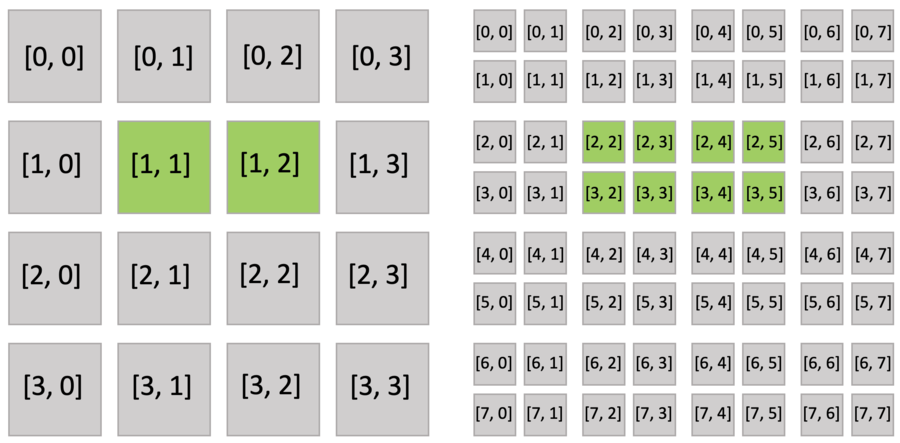Executing the `activate()` function automatically activates `block[1,1]`, which includes `x[2,3]`, and `block[1,2]`, which includes `x[2,4]`. Other pixels of `block[1,1]` (`x[2,2], x[3,2], x[3,3]`) and `block[1,2]` (`x[2,5], x[3,4], x[3,5]`) are also implicitly activated because all pixels in the dense block share the same activity value.

In fact, the sparse field is an SNode tree shown in the following figure. You can use the struct-for loop to loop over the different levels of the SNode tree like the `print_active()` function in `pointer.py`. `for i, j in block` would loop over all active `pointer` SNodes. `for i, j in pixel` would loop over all active `dense` SNodes.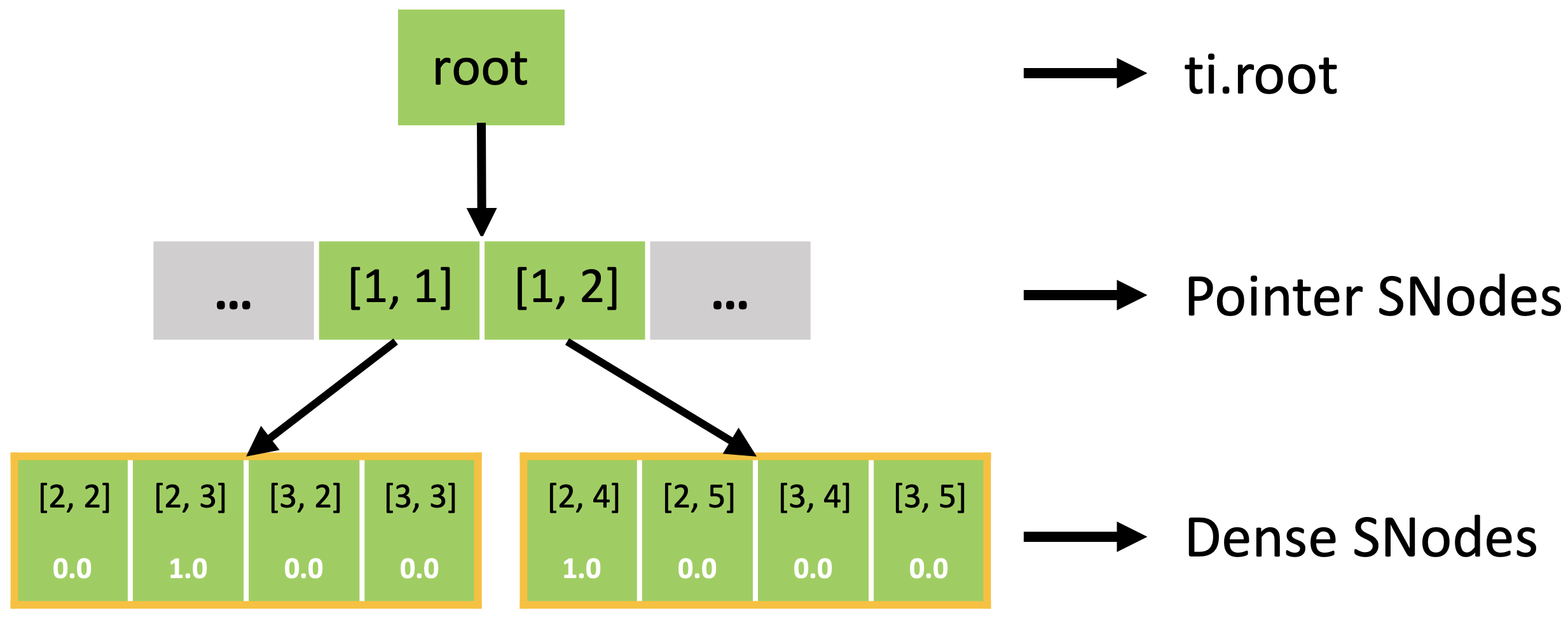While a null pointer can effectively represent an empty sub-tree, at the leaf level using 64 bits to represent the activity of a single pixel can consume too much space. For example, if each pixel contains a single `f32` value (4 bytes), the 64-bit pointer pointing to the value would take 8 bytes. The fact that storage costs of pointers are higher than the space to store the value themselves goes against our goal to use sparse spatial data structures to save space.

To amortize the storage cost of pointers, you could organize pixels in a blocked manner and let the pointers directly point to the blocks like the data structure defined in `pointer.py`.

One caveat of this design is that pixels in the same `dense` block can no longer change their activity flexibly. Instead, they share a single activity flag. To address this issue, the `bitmasked` SNode additionally allocates 1-bit per pixel data to represent the pixel activity.

``x = ti.field(ti.f32)block = ti.root.pointer(ti.ij, (4,4))pixel = block.bitmasked(ti.ij, (2,2))pixel.place(x)@ti.kerneldef activate():    x[2,3] = 1.0    x[2,4] = 2.0@ti.kerneldef print_active():    for i, j in block:        print("Active block", i, j)    for i, j in x:        print('field x[{}, {}] = {}'.format(i, j, x[i, j]))``

The code snippet above also creates an 8x8 sparse grid. The only difference between `bitmasked.py` and `pointer.py` is that the bitmasked SNode replaces the dense SNode (line 3). As shown in the figure below, the active blocks are the same as `pointer.py`. However, the bitmasked pixels in the block are not all activated, because each of them has an activity value.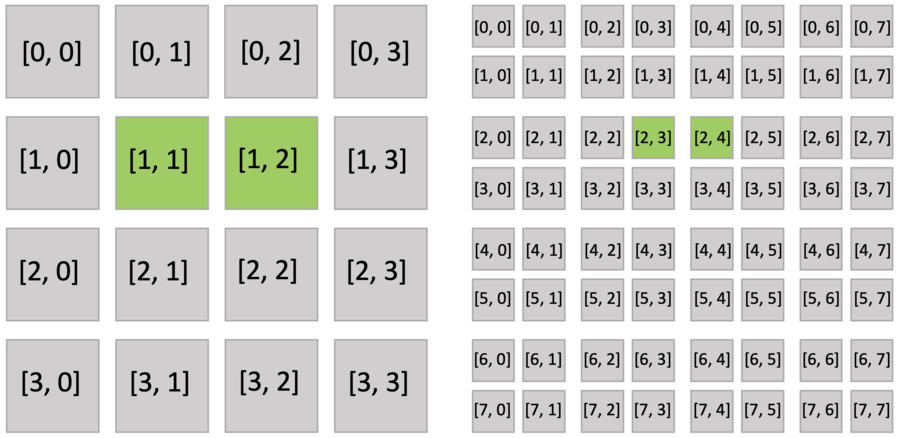The bitmasked SNodes are like dense SNodes with auxiliary activity values.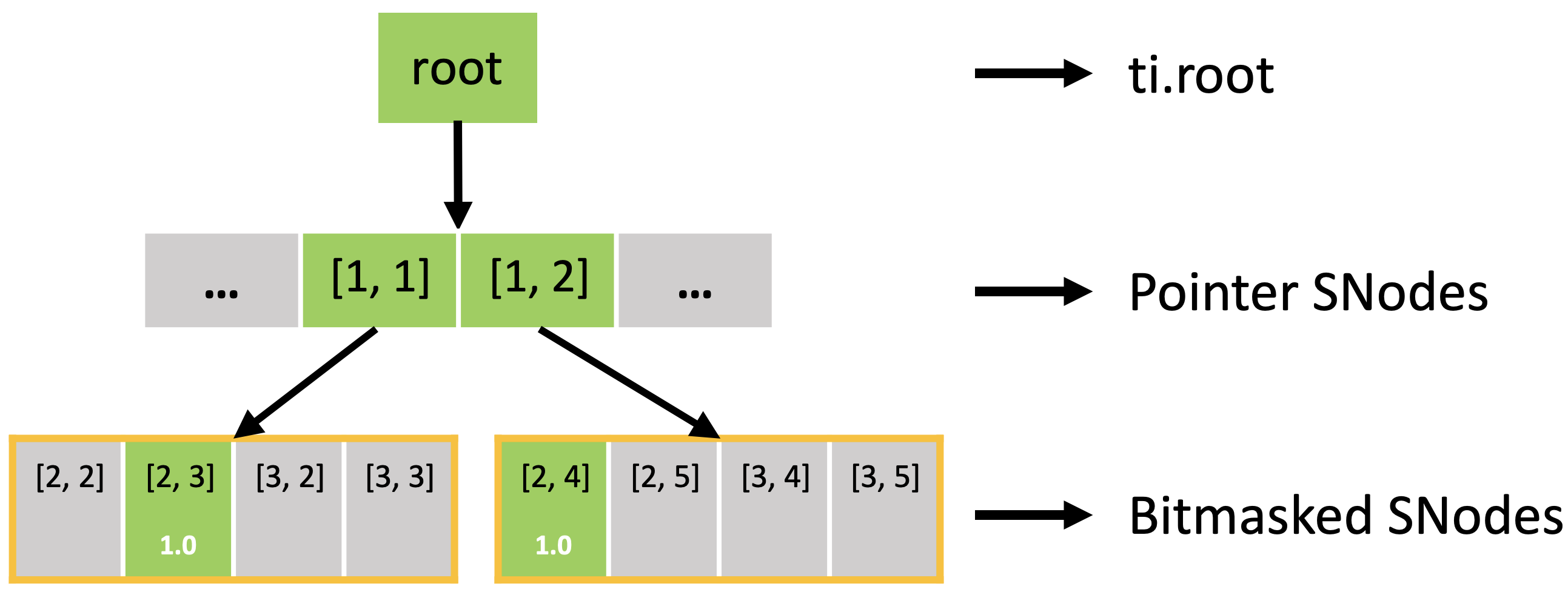### Dynamic SNode

To support variable-length fields, Taichi provides dynamic SNodes. The code snippet below first creates a 5x1 dense block (line 2). Then each cell of the dense block contains a variable-length dynamic container (line 3). The maximum length of the dynamic container is 5. In the `make_lists()` function, you can use `ti.append()` to add a value to the end of a dynamic SNode. `x.parent()` is the same as `pixel`. The dense field `l` stores the length of each dynamic SNode.

dynamic.py
``x = ti.field(ti.i32)block = ti.root.dense(ti.i, 5)pixel = block.dynamic(ti.j, 5)pixel.place(x)l = ti.field(ti.i32)ti.root.dense(ti.i, 5).place(l)@ti.kerneldef make_lists():    for i in range(5):        for j in range(i):            ti.append(x.parent(), i, j * j)  # ti.append(pixel, i, j * j)        l[i] = ti.length(x.parent(), i)  # [0, 1, 2, 3, 4]``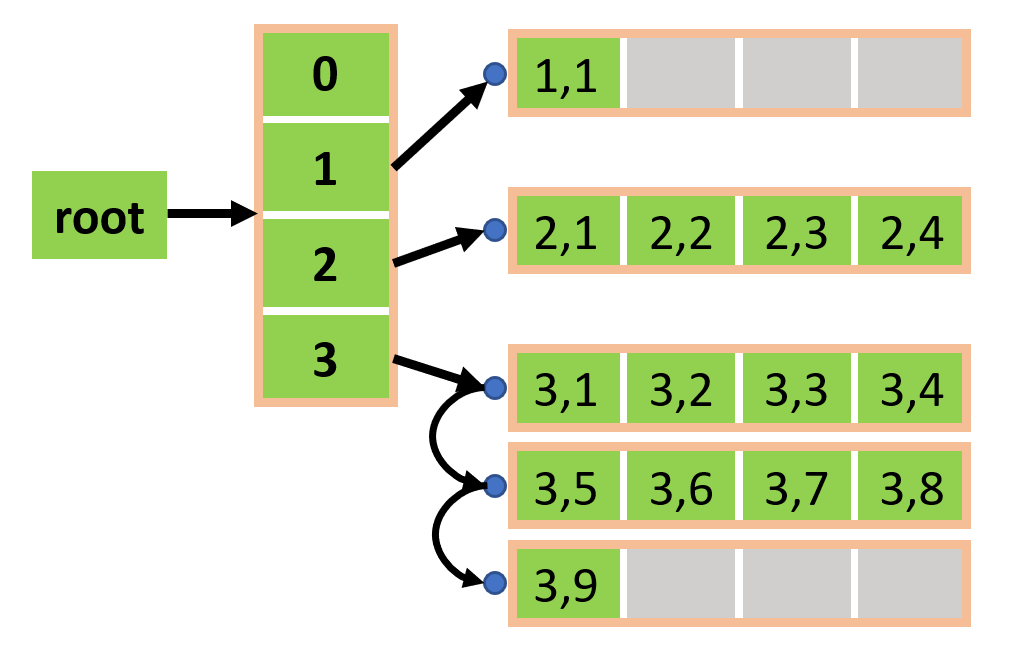## Computation on sparse spatial data structures

### Sparse struct-fors

Efficiently looping over sparse grid cells that distribute irregularly can be challenging, especially on parallel devices such as GPUs. In Taichi, struct-fors natively support sparse spatial data structures and only loop over currently active pixels with automatic efficient parallelization.

### Explicitly manipulating and querying sparsity

Taichi also provides APIs that explicitly manipulate data structure sparsity. You can manually check the activity of a SNode, activate a SNode, or deactivate a SNode. We now illustrate these functions based on the field defined below.

``x = ti.field(dtype=ti.i32)block1 = ti.root.pointer(ti.ij, (3, 3))block2 = block1.pointer(ti.ij, (2, 2))pixel = block2.bitmasked(ti.ij, (2, 2))pixel.place(x)``

#### 1. Activity checking

You can use `ti.is_active(snode, [i, j, ...])` to explicitly query if `snode[i, j, ...]` is active or not.

``@ti.kerneldef activity_checking(snode: ti.template(), i: ti.i32, j: ti.i32):    print(ti.is_active(snode, [i, j]))for i in range(3):    for j in range(3):        activity_checking(block1, i, j)for i in range(6):    for j in range(6):        activity_checking(block2, i, j)for i in range(12):    for j in range(12):        activity_checking(pixel, i, j)``

#### 2. Activation

You can use `ti.activate(snode, [i, j, ...])` to explicitly activate a cell of `snode[i, j, ...]`.

``@ti.kerneldef activate_snodes()    ti.activate(block1, [1, 0])    ti.activate(block2, [3, 1])    ti.activate(pixel, [7, 3])activity_checking(block1, [1, 0]) # output: 1activity_checking(block2, [3, 1]) # output: 1activity_checking(pixel, [7, 3])  # output: 1``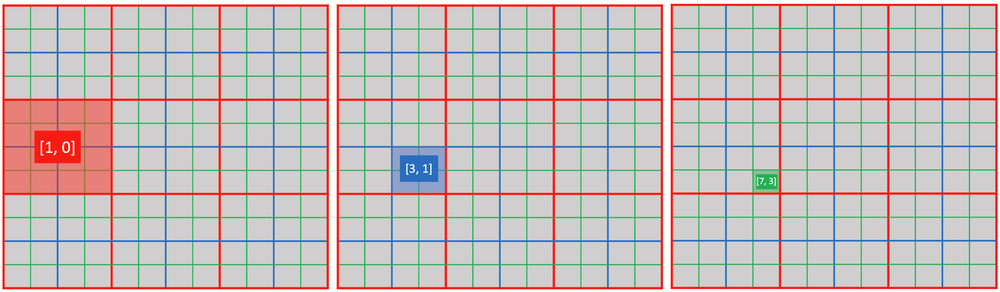#### 3. Deactivation

• Use `ti.deactivate(snode, [i, j, ...])` to explicitly deactivate a cell of `snode[i, j, ...]`.
• Use `snode.deactivate_all()` to deactivate all cells of SNode `snode`. This operation also recursively deactivates all its children.
• Use `ti.deactivate_all_snodes()` to deactivate all cells of all SNodes with sparsity.

When deactivation happens, the Taichi runtime automatically recycles and zero-fills memory of the deactivated containers.

##### note

For performance reasons, `ti.activate(snode, index)` only activates `snode[index]`. The programmer must ensure that all ancestor containers of `snode[index]` is already active. Otherwise, this operation results in undefined behavior.

Similarly, `ti.deactivate` ...

• does not recursively deactivate all the descendants of a cell.
• does not trigger deactivation of its parent container, even if all the children of the parent container are deactivated.

#### 4. Ancestor index query

You can use `ti.rescale_index(descendant_snode/field, ancestor_snode, index)` to compute the ancestor index given a descendant index.

``print(ti.rescale_index(x, block1, ti.Vector([7, 3]))) # output: [1, 0]print(ti.rescale_index(x, block2, [7, 3]))            # output: [3, 1]print(ti.rescale_index(x, pixel,  [7, 3]))            # output: [7, 3]print(ti.rescale_index(block1, block2, [3, 1]))       # output: [1, 0]``

Regarding line 1, you can also compute the `block1` index given `pixel` index `[7, 3]` as `[7//2//2, 3//2//2]`. However, doing so couples computation code with the internal configuration of data structures (in this case, the size of `block1` containers). By using `ti.rescale_index()`, you can avoid hard-coding internal information of data structures.